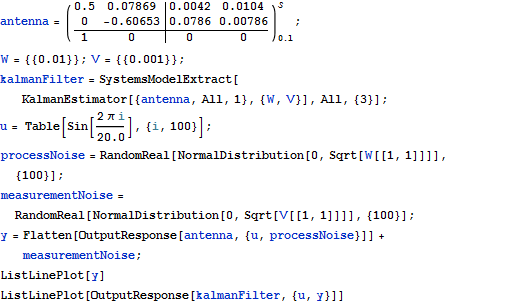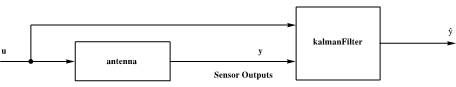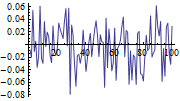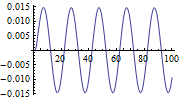# Control Systems

Since Mathematica 8, controls engineers can compute, analyze, and dynamically present control systems now. Besides comprehensive numeric methods, the symbolic features provide unexpected and uprecedented possibilities to simulate control tasks.

• State-space models
• Control systems with built-in symbolic computation capabilities

The components of these control system packages support computation in mechanics, electrical engineering, chemistry, aeronautical engineering, biology, and economics.
Analyze and design controls using classical techniques and state spaces, develop solutions for analog and digital systems, and simulate models in configurations with open and closed loops.

Some examples of control systems:

## Connect Two Systems in Parallel

Obtain the equivalent input-output model by connecting two systems in parallel.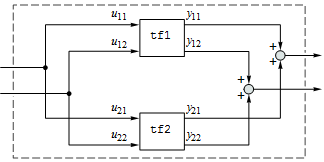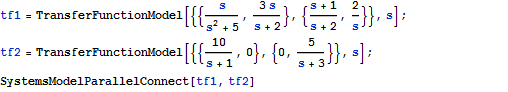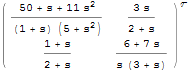## Interactively Analyze System Behavior

Determine critical points of system behavior, such as break-away, break-in, and imaginary-axis crossings, using an interactive root-locus plot.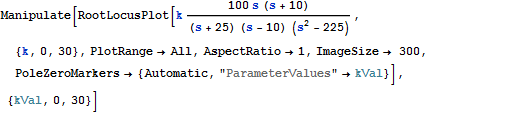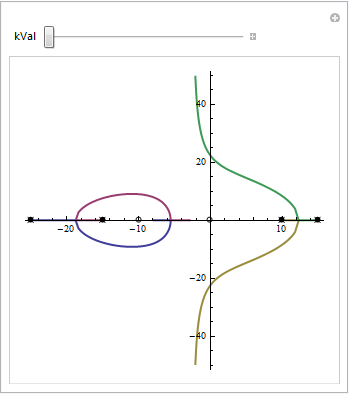## Determine System Stability Using Built-in Functions

Analyze a system's stability from its Nyquist plot.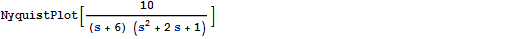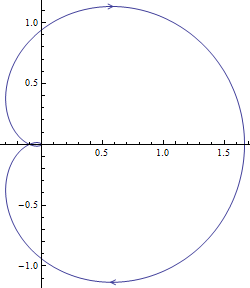## Visualize the Relative Stability of Systems

Gain and phase margins in a Bode plot.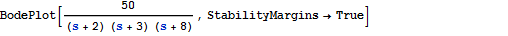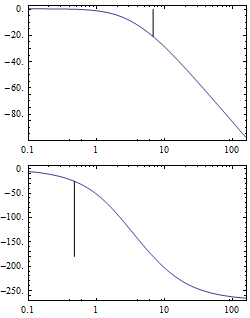## Study the Frequency Response of a Multivariable Systems

The singular value plot of a transfer-function model.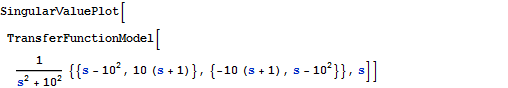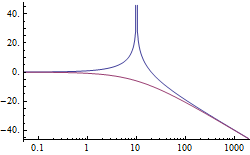## Build Regulators and Observers for Systems

The trajectories of the states and a Luenberger observer's state estimates.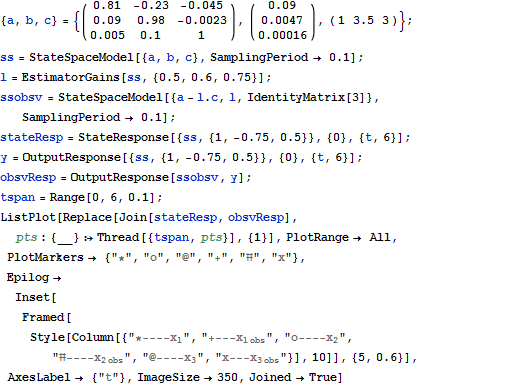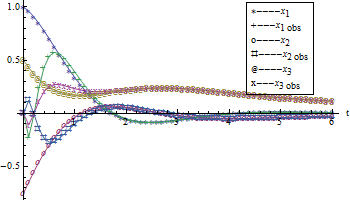## Simulate the Response of State-Space or Transfer-Function Models

The step responses of a second-order system for different values of damping.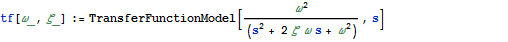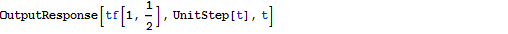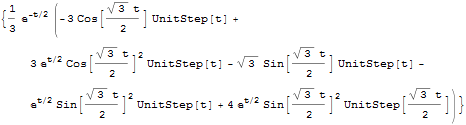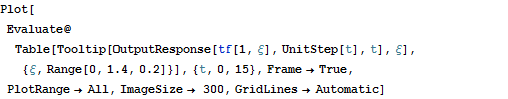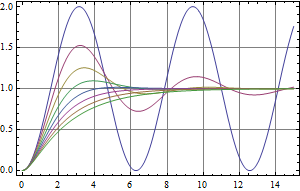## Construct a Kalman Filter for a Stochastic System

Output of a system before and after optimal filtering.